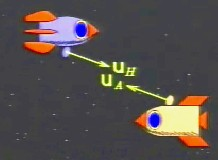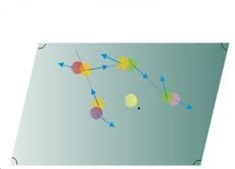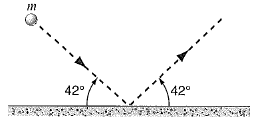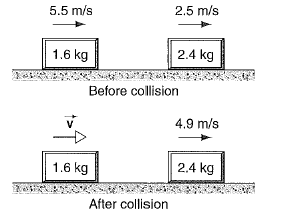Click to Chat

1800-1023-196

+91-120-4616500

CART 0

• 0

MY CART (5)

Use Coupon: CART20 and get 20% off on all online Study Material

ITEM
DETAILS
MRP
DISCOUNT
FINAL PRICE
Total Price: Rs.

There are no items in this cart.
Continue Shopping• Complete Physics Course - Class 11
• OFFERED PRICE: Rs. 2,968
• View Details

Momentum

Table of Content

Quantitative Definition of Linear Momentum

Non-Relativistic Momentum

Relativistic Momentum

Newton’s Second Law of Motion and Momentum

Momentum of a System of Particles

Related ResourcesMomentum of a body is defined as the amount of motion contained in a body.

Quantity of motion or the momentum of the body depends upon,

(a) mass of the body.

(b) velocity of the body.

Therefore momentum of a body of mass ‘m’ and velocity ‘v’ will be,

Quantitative Definition of Linear Momentum

Momentum of a body is equal to the product of its mass and velocity. Momentum is a vector quantity and possesses the direction of velocity.Units:-

S.I:- kg m s-1

C.G.S:- g cm s-1

Momentum can be put into following two categories.

Dimension:-

[MLT-1]

(a) Non-Relativistic Momentum

According to classical physics (or non-relativistic physics) which is based upon the concepts of Newton’s laws of motion, mass of a body is considered to be a constant quantity, independent of the velocity of body. In that case momentum  is given by,

.

Thus, momentum of a body is a linear function of its velocity.

(b) Relativistic MomentumIn accordance to Einstein’s special theory of relativity, mass of a body depends upon the relative velocity ‘v’ of the body with respect to the observer. If ‘m0’ is the mass of body observed by an observer at rest with respect to body, its relativistic mass ‘m’ is given by,

Therefore, momentum of a body according to the concepts of theory of relativity is given by,

Thus, relativistic momentum is not a linear function of v.

Newton’s Second Law of Motion and MomentumThe rate of change of momentum of a body is proportional to the resultant external force acting on the body and is the direction of that force.

Newton’s second law provides a quantitative definition of the force.

Let  be the instantaneous velocity of the body. Momentum  of the body is given by,

According to second law,

∝ (rate of change of momentum)

Or,

Or,

Or,

Here ‘k’ is the constant of proportionality. Mass ‘m’ of a body is considered to be a constant quantity.

or,

The units of force are also selected that ‘k’ becomes one.

Thus, if a unit force is chosen to be the force which produces a unit acceleration in a unit mass,

i.e., F = 1, m = 1 and a = 1.

Then, k = 1

So, Newton’s second law can be written , in mathematical form, as

i.e., Force = (mass) (acceleration)

Momentum of a System of ParticlesConsider a system of n particles with masses m1,m2 etc. Each with its own velocity, and linear momentum. The particle may interact with each other, and external forces may act on them as well. The system as a whole has a total linear momentum P, which is defined to be the vector sum of the individual particle linear momenta.

Here we assume that total mass M = ∑i mi of the system remains constant with time. If we assume that each particle will have some momentum then the system as a whole will have momentum P given by,

P = p1 + p2 + p3 + . . . + pn

If we compare this equation with

vcm= (m1v1 + m2v2+ . . . + mnvn)/ M,

We see that,

P = Mvcm

Which gives us another way to define the linear momentum of a system of particles.

The linear momentum of a system of particles is equal to the product of the total mass M of the system and the velocity of the center of mass.

Taking the time derivative of this equation and comparing with F = Macm, we can write Newton's second law for a system of particles in the equivalent form

Table below displays the important relations that we have derived for single particles and for systems of particles.

Problem 1:-

A 4.88-kg object with a speed of 31.4 m/s strikes a steel plate at an angle of 42.0º and rebounds at the same speed and angle (Below Figure). What is the change (magnitude and direction) of the linear momentum of the object?Concept:-

Momentum of the object (p) is defined as the mass of the object (m) time’s velocity of the object (v).

p = mv      …… (1)

Solution:-

The below figure shows a 4.88-kg object with a speed of 31.4 m/s strikes a steel plate at an angle of 42.0° and rebounds at the same speed with same angle which is shown geometrically.

The initial momentum of the object is pi when it strikes the steel plate.

To find initial momentum pi, substitute pi for p, 4.88-kg for mass m of the object and 31.4 m/s for velocity of the object in the equation p = mv,

pi = mv

= (4.88 kg) (31.4 m/s)

= 153 kg. m/s        …… (2)

Since the final momentum pf of the object is equal to the initial momentum pi of the object, therefore,

pf = 153 kg. m/s      …… (3)

In the above figure the angle θ will be,

θ = 42º + 42º

= 84º

Using cosine law, the magnitude of change of the linear momentum of the object Δp will be,

Δp = √( pi)2 + (pf)2 + 2 (-pi)(pf)cos θ     …… (4)

To obtain magnitude of linear momentum Δp, substitute 153 kg. m/s for pi, 153 kg. m/s for pf and 84° for the angle θ in the equation Δp = √( pi)2 + (pf)2 + 2 (-pi)(pf) cos θ,

Δp = √( pi)2 + (pf)2 + 2 (-pi)(pf) cos θ

= √(153 kg. m/s)2 + (153 kg. m/s)2 - 2 (153 kg. m/s)( 153 kg. m/s) cos 84°

= 205 kg. m/s

From the above observation we conclude that, the change of the linear momentum of the object will be, 205 kg. m/s and also from the figure the direction of change of the linear momentum of the object is perpendicular to the steel plate.

Problem 2:-

A 2000-kg truck travelling north at 40.0 km/h turns east and accelerates to 50.0 km/h. What is the magnitude and direction of the cahnge of the truck’s momentum?

Concept:-

Momentum of the body p is equal to the mass of the body m times velocity of the body v.

So, p = mv

Initial momentum pi of the truck having initial velocity vi will be,

pi = mvi

and the final momentum pf of the truck having final velocity vf will be,

pf = mvf

Momentum is a vector quantity. If Δpx is the x-component of momentum p and Δpy is the y-component of momentum p, then the angle θ between Δpx and Δpy will be,

θ = tan-1(Δpy/ Δpx)

Solution:-

To obtain the Initial momentum pi of the truck having initial velocity vi, substitute 2000 kg for m, 40 km/h for vi in the equation pi = mvi,

pi = mvi

= (2000 kg) (40 km/h)

= 8.00×104 kg.km/h

So, pi = 8.00×104 kg.km/h j   (j for north direction)

To obtain the final momentum pf of the truck having final velocity vf, substitute 2000 kg for m, 50 km/h for vi in the equation pf = mvf,

pf = mvf

= (2000 kg) (50 km/h)

= 1.00×105 kg.km/h

So, pf = 1.00×105 kg.km/h i    (i for east direction)

Thus, Δp = pf - pi

= (1.00×105 kg.km/h i) – (8.00×104 kg.km/h j)

So the magnitude of Δp will be,

Δp = √(Δpx)2 +(Δpy)2

= √ (1.00×105 kg.km/h)2 +(8.00×104 kg.km/h)2

= 1.28 ×105 kg.km/h

To obtain the direction, substitute 8.00×104 kg.km/h for Δpy and 1.00×105 kg.km/h for Δpx in the equation θ = tan-1(Δpy/ Δpx),

θ = tan-1(Δpy/ Δpx)

= tan-1[(8.00×104 kg.km/h) /( 1.00×105 kg.km/h)]

=38.7 ° (south of east)

From the above observation we conclude that, the magnitude of the change of the truck’s momentum will be 1.28 ×105 kg.km/h and the direction will be 38.7 ° (south of east).

Problem 3:-

The block in below figure slides without friction. what is the velocity v of the 1.6-kg block after the collision?Concept:-

Momentum of the body p is equal to the mass of the body m times velocity of the body v.

So, p = mv

In accordance to the principle of conservation of energy, the final momentum of the system is equal to the initial momentum of the system.

Consider the initial momentum of the block 1 is pi,1, initial momentum of the block 2 is pi,2, final momentum of the block 1 is pf,1 and final momentum of the block 2 is pf,2.

To obtain the initial momentum pi,1 of the block 1, substitute pi,1 for p, m1 for the mass of the block 1 and v1,i for the initial velocity of the block 1in the equation p = mv,

p = mv

pi,1 = m1 v1,i

To obtain the initial momentum pi,2 of the block 2, substitute pi,2 for p, m2 for the mass of the block 2 and v2,i for the initial velocity of the block 2 in the equation p = mv,

p = mv

pi,2 = m2 v2,i

To obtain the final momentum pf,1 of the block 1, substitute pf,1 for p, m1 for the mass of the block 1 and v1,f for the final velocity of the block 1in the equation p = mv,

p = mv

pf,1 = m1 v1,f

To obtain the final momentum pf,2 of the block 2, substitute pf,2 for p, m2 for the mass of the block 2 and v2,f for the final velocity of the block 2 in the equation p = mv,

p = mv

pf,2 = m2 v2,f

Solution:-

So applying conservation of momentum to this system, the sum of the initial momentum of the block 1 and block 2 will be equal to the sum of the final momentum of the block 1 and block 2.

pf,1 + pf,2 = pi,1 + pi,2

Substitute, m1 v1,f for pf,1, m2 v2,f for pf,2, m1 v1,i for pi,1 and m2 v2,i for pi,2 in the equation pf,1 + pf,2 = pi,1 + pi,2,

pf,1 + pf,2 = pi,1 + pi,2

m1 v1,f + m2 v2,f = m1 v1,i + m2 v2,i

m1 v1,f = m1 v1,i + m2 (v2,i - v2,f)

So,

v1,f = v1,i + m2/ m1 (v2,i - v2,f)

To obtain the velocity v of the 1.6-kg block after the collision (v1,f), substitute 5.5 m/s for v1,i, 2.4 kg for m2 and 1.6 kg for m1, 2.5 m/s for v2,i and 4.9 m/s for v2,f in the equation  v1,f = v1,i + m2/ m1 (v2,i - v2,f),

v1,f = v1,i + m2/ m1 (v2,i - v2,f)

= (5.5 m/s) + (2.4 kg)/(1.6 kg) [(2.5 m/s) – (4.9 m/s)]

= 1.9 m/s

From the above observation we conclude that, the velocity v of the 1.6-kg block after the collision (v1,f) would be 1.9 m/s.

Problem 4:-

A golfer hits a golf ball, imparting to it an initial velocity of magnitude 52.2 m/s directed 30º above the horizontal. Assuming that the mass of the ball is 46.0 g and the club and ball are in contact for 1.20 ms, find (a) the impulse imparted to the ball, (b) the impulse imparted to the club, and (c) the average force exerted on the ball by the club.

Concept:-

The impulse of the net force J acting on a particle during a given time interval is equal to the change in momentum Δp of the particle during that interval.

So, J = Δp

= pi – pf

Here pf is the final momentum and pi is the initial momentum of the body.

Force (F) is equal to the rate of change of momentum (Δp) body.

Therefore the average force will be,

F = Δp/ Δt

Solution:-

The initial momentum pi of the ball will be,

pi = mvi

To obtain the initial momentum pi of the ball, substitute 46.0 g for the mass of the ball and 52.2 m/s for vi in the equation pi = mvi,

pi = mvi

= (46.0 g) (52.2 m/s)

= (46.0 g×10-3 kg/1 g) (52.2 m/s)

= 2.4 kg.m/s

= (2.4 kg.m/s) (1 N/1 kg.m/s2)

= 2.4 N.s

As the final speed of the ball is zero, thus the final momentum pf of the ball will be zero.

So, pf = 0

To find out the impulse J imparted to the ball, substitute 2.4 N.s for pi and 0 for pf in the equation J = pi – pf,

J = Δp

= pi – pf

= 2.4 N.s -0

= 2.4 N.s

Therefore the impulse J imparted to the ball would be 2.4 N.s.

b) The impulse J imparted to the club is just opposite that imparted to the ball. Therefore the impulse J imparted to the club will be 2.4 N.s.

c) To obtain the average force F exerted on the ball by the club, substitute 2.4 N.s for Δp and 1.20 ms for t in the equation F = Δp/ Δt,

F = Δp/ Δt

= 2.4 N.s/1.20 ms

= (2.4 N.s)/((1.20 ms)(10-3 s/1ms))

= 2000 N

From the above observation we conclude that, the average force F exerted on the ball by the club would be 2000 N.

Related Resources

Look into the Sample Papers of Previous Years to get a hint of the kinds of questions asked in the exam.

You can get the knowledge of Useful Books of Physics.### Course Features

• 101 Video Lectures
• Revision Notes
• Previous Year Papers
• Mind Map
• Study Planner
• NCERT Solutions
• Discussion Forum
• Test paper with Video Solution